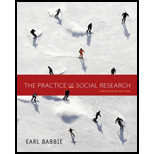Chapter 6, Problem 3RQE### The Practice of Social Research (M...

14th Edition
Earl R. Babbie
ISBN: 9781305104945

#### Solutions

Chapter
Section### The Practice of Social Research (M...

14th Edition
Earl R. Babbie
ISBN: 9781305104945
Textbook Problem

# Make up three questionnaire items that measure attitudes toward nuclear power and that would probably form a Guttman scale.

Summary Introduction

Introduction:

Guttman scale requires binary outcomes that can be ranked. If a person can answer the third question, they should be able to answer the two previous questions, which are in order of difficulty.

1. Which type of power plant emits more pollution, coal or nuclear?

2. Which of these is the primary exhaust emitted by nuclear power plants, steam or radiation?

3. How are spent nuclear fuel rods stored, in underground bunkers, or landfills?

Explanation

(This question requires a subjective answer, this is an example.) A questionnaire to determine attitudes toward nuclear power would need to be much longer than three items. These are simply a sample of the type of items that could lead to a Guttman scale, which uses closed-ended questions to generate scores from questions of increasing difficulty.

1. Which type of power plant emits more pollution, coal or nuclear?

a. This is the simplest item of the set, used to determine the respondent’s knowledge of the relative levels of pollution produced in these modes of power generation...

### Still sussing out bartleby?

Check out a sample textbook solution.

See a sample solution

#### The Solution to Your Study Problems

Bartleby provides explanations to thousands of textbook problems written by our experts, many with advanced degrees!

Get Started

## Chapter 6 Solutions